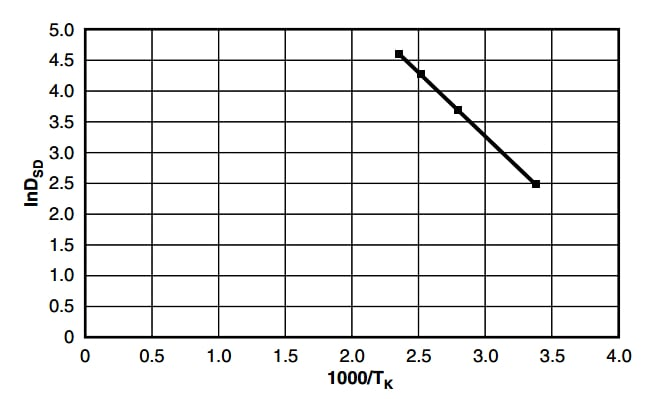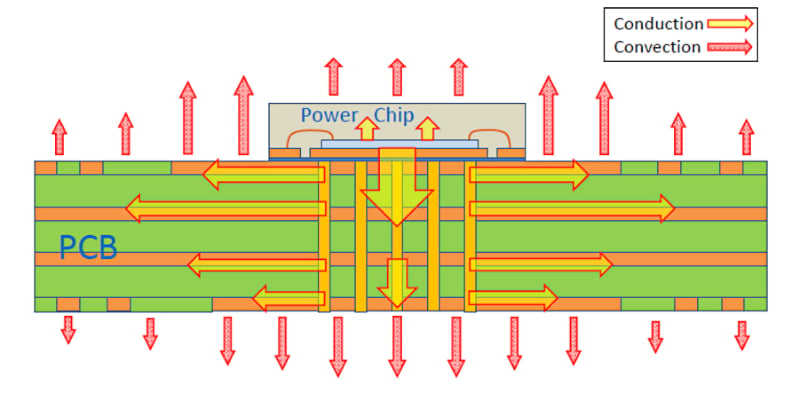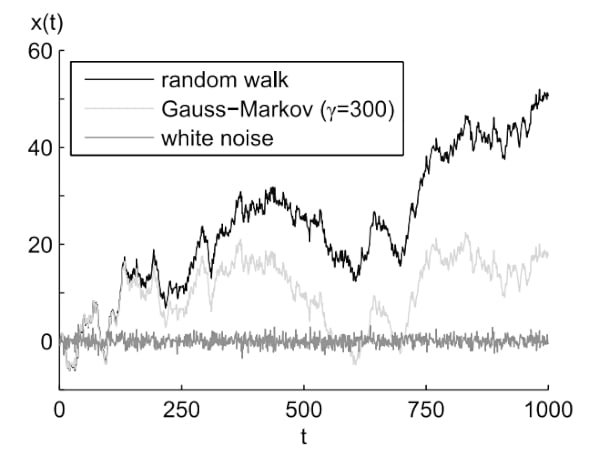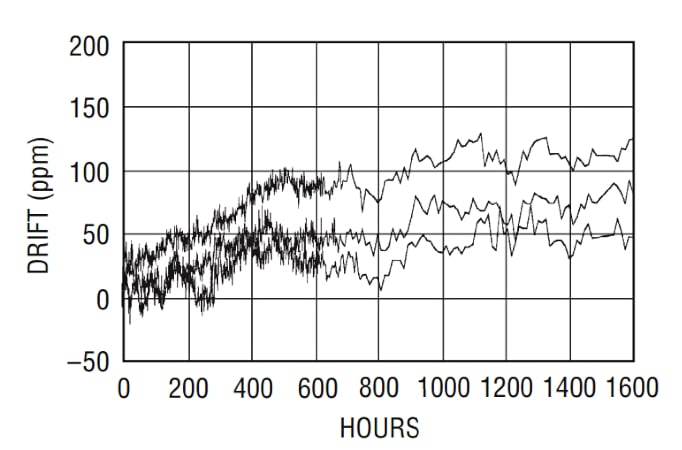Technical Article

# Electronic Component Aging—the Aging Effects of Resistors and Op-amps

August 28, 2022 by Dr. Steve Arar

## Learn about the aging behavior of resistors and amplifiers using temperature calculations and the Arrhenius equation to understand resistor drift, resistor stability, and op-amp drift.

Previously, we discussed the high-temperature accelerated aging method for evaluating the long-term stability of the electronic components using relatively shorter test durations.

In this article, we’ll continue this discussion and take a look at the aging behavior of resistors and amplifiers.

### Aging Prediction—Resistor Drift Due to Aging

To begin with, let's remember that the value of a resistor changes with time. In many circuits, only a gross level of precision is required and the resistor aging might not be a serious issue. However, certain precision applications require resistors with a long-term drift as low as a few parts per million over the specified lifetime. Therefore, it is important to develop aging prediction models with sufficient accuracy to ensure that the employed precision resistors maintain the specified precision over the entire lifetime of the system. One company, Vishay, suggests using the following equation (Equation 1) to calculate the long-term variation of a thin-film resistor:

$$\frac{\Delta R}{R}(t,\theta_{j}) = 2^{\frac{\theta_{j}-\theta_{0}}{30\,K}}\,\times \sqrt{\frac{t}{t_{0}}}\times\,\frac{\Delta R}{R}(t_{0},\theta_{0})$$

##### Equation 1.

Where:

$$\frac{\Delta R}{R}(t_{0},\theta_{0})$$

Is the reference drift of the resistor at the reference time $$t_{0}$$ and temperature $$\theta_{0}$$.

While:

$$\frac{\Delta R}{R}(t,\theta_{j})$$

Is the drift value after the desired operating time of the resistor, t, at temperature $$\theta_{j}$$.

Equation 1 shows that raising the resistor's operating temperature by 30 °K increases its long-term drift by a factor of 2. Additionally, the drift increases with the cube root of the operation time. For example, if the 1000-hour drift of the resistor at 125 °C is less than 0.25%, the resistor drifts after 8000 hours of operation at the same temperature $$(\theta_{j}=\theta_{0})$$ is estimated by:

$$\frac{\Delta R}{R}(t= 8000\,h) = \sqrt{\frac{8000}{1000}} \times\frac{\Delta R}{R}(t=1000\,h)\leq 2\times 0.25\% = 0.5\%$$

### Arrhenius Equation for Resistor Aging Prediction

In Equation 1, the term that takes the temperature dependence into account is derived from the Arrhenius rate law which is also repeated below as Equation 2:

$$Process \text{ } Rate\text{ }(PR) = Ae^{-\frac{E_a}{K_BT}}$$

##### Equation 2.

This equation specifies how the speed of a reaction changes with temperature in Kelvin (T). According to Vishay, the aging process of both thin-film and foil resistors obeys the Arrhenius equation. Figure 1 shows the aging data of identical foil resistors at different temperatures.##### Figure 1. Image used courtesy of Vishay

In this figure, the natural logarithm of the standard deviation of the resistors’ drift distribution (Ln(DSD)) is plotted against $$\frac{1000}{T}$$.

Note that a straight line can be fit to these data points. This is consistent with the Arrhenius equation, which can be expressed as:

$$Ln(PR)=Ln(A)-\frac{E_a}{k_B}\times \frac{1}{T}$$

This equation shows that the plot of Ln(PR)versus $$\frac{1}{T}$$ is a straight line when a reaction obeys the Arrhenius equation.

Since this relationship holds true for the data points in Figure 1, we can conclude that the aging process of these resistors obeys the Arrhenius law.

### Estimate Resistor Temperature—Improving Long-term Resistor Stability

Based on Equation 1, keeping the resistor at a lower temperature can reduce its drift with time. The remaining question is, how can we keep the resistor cooler?

The θ terms in Equation 1 refer to the resistor temperature rather than the ambient temperature. The resistor temperature (θ Resistor) can be estimated by the following equation:

$$\theta_{Resistor}=\theta_{A}+P\times R_{th}$$

Where:

• θA is the ambient temperature
• Rth is the thermal resistance of the resistor
• P is the power dissipated in the resistor

This equation shows that, in addition to the ambient temperature, the heat dissipated in the resistor and the thermal resistance value can affect the resistor temperature. For the resistor to run cooler, we can limit the power dissipated in the resistor if possible. Besides, changing the characteristics of the PC board, such as the trace density and the number of the power/ground planes, can change the value of the effective thermal resistance of the system. This change is because the PC board acts as a heatsink soldered to the resistor. A more efficient heatsink can improve heat transfer and keep the circuit components, including the precision resistors, cooler.

Figure 2 shows how heat flows through the PCB and the package case of a typical IC.##### Figure 2. Image used courtesy of onsemi

Adjusting different design parameters, we can attempt to keep the resistor temperature below a typical maximum value of 85 °C to achieve improved long-term stability.

It is also worth mentioning that operating a resistor at power levels higher than the nominal value can lead to a long-term drift larger than that predicted by the Arrhenius-based equations. Above the rated power, some hot spots can appear in parts of the resistive material where the aging process is accelerated. This can lead to a drift value larger than that predicted by the average temperature of the resistor.

### Op-amp Aging Effects and Long-term Op-Amp Drift

The input offset voltage of an amplifier also changes due to aging. This can produce an error that varies with time and limit the minimum DC signal that can be measured. While the offset drift with temperature for a typical general purpose precision op-amp is in the range of 1–10 μV/°C, the op-amp offset variation caused by aging is about a few μV during the first 30 days of operation.

We discussed that the long-term drift of a resistor increases with the cube root of its operation time and the crystal aging tends to have a logarithmic relationship with time. Deviations of the op-amp offset voltage due to aging are also a nonlinear function of time. The long-term drift in the op-amp offset is proportional to the square root of the elapsed time. Therefore, if the aging effect is specified to be 1 μV/1000 hours, the offset can change by about 3 μV/year as calculated below:

$$Drift(t=8760\,hours) = Drift(t=1000\,hours)\times\sqrt{\frac{8760}{1000}} \simeq2.96\frac{\mu V}{year}$$

The long-term variation in offset is generally specified in μV/month or μV/1000 hours.

### The Random Walk Phenomenon: Electronic Component Aging is a Random Process

It is important to note that the aging effect is a random process and the real aging behavior of a device can be too complex to describe with a simple formula. Aging is sometimes considered to be a “random walk" phenomenon. The random walk process results when uncorrelated random “steps” are integrated. Its discrete time representation is given by:

$$x_{k}=x_{k-1}+w_{k}$$

Where:

• xk and xk-1 are two consecutive samples of the random process (aging effect in our discussion)
• wis white noise

Figure 3 below shows an example of white noise along with the random walk that is obtained from this same white noise.##### Figure 3. Image used courtesy of Handbook of Signal Processing Systems

With a random walk process, the more steps we integrate, the more likely we are to move away from the initial value. A similar trend is observed in the aging data gathered from electronic components. For example, compare the above random walk process in Figure 3 with the measured long-term drift of the LT1461 at 30 °C shown in Figure 4 below.##### Figure 4. Long-term drift graph for the LT1461. Image used courtesy of Linear Technology

If a zero-mean white noise is used to generate a random walk process, the average difference between two arbitrary samples [video] of the random walk process will be proportional to the square root of the time difference between the two samples. This is consistent with the simple equation we discussed above for modeling the long-term drift of the op-amp offset voltage where the drift was assumed to be proportional to the square root of the elapsed time.

Random walks can be important processes and show up in various other scientific and social disciplines. For example, a random walk process can model part of the noise that appears at the output of a MEMS gyroscope. In the next article in this series, we’ll examine the aging behavior of voltage references.